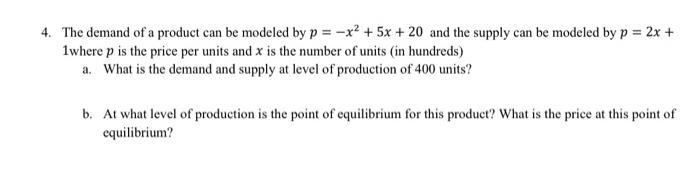Home / Expert Answers / Calculus / 4-the-demand-of-a-product-can-be-modeled-by-p-x2-5x-20-and-the-supply-can-be-modeled-by-p-2x-1-pa691

# (Solved): 4. The demand of a product can be modeled by p=x2+5x+20 and the supply can be modeled by p=2x+ 1 ...4. The demand of a product can be modeled by and the supply can be modeled by 1where is the price per units and is the number of units (in hundreds) a. What is the demand and supply at level of production of 400 units? b. At what level of production is the point of equilibrium for this product? What is the price at this point of equilibrium?

We have an Answer from Expert